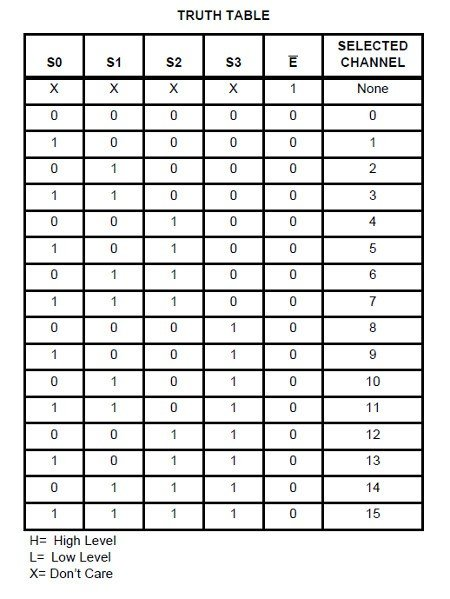# ESP32 and 4067 led example

The 4067 was one of the IC’s that I wanted to connect up to various microcontrollers as it looke dextremely useful, here is a quick description from one of the datasheets with an overview of the functionality

The HEF4067B is a 16-channel analog multiplexer/demultiplexer with four address inputs (A0 to A3), an active LOW enable input (E), sixteen independent inputs/outputs (Y0 to Y15) and a common input/output (Z). The device contains sixteen bidirectional analog switches, each with one side connected to an independent input/output (Y0 to Y15) and the other side connected to the common input/output (Z). With E LOW, one of the sixteen switches is selected (low-impedance ON-state) by A0 to A3. All unselected switches are in the high-impedance OFF-state. With E HIGH all switches are in the high-impedance OFF-state, independent of A0 to A3. The analog inputs/outputs (Y0 to Y15 and Z) can swing between VDD as a positive limit and VSS as a negative limit. VDD to VSS may not exceed 15 V.

Here is the pinout of the 4067You can see depending on the state of pins S0 – S3 determines the output channel pin that is used. So if S0 to S3 are 0 then Channel 0 which is Pin 9 is selectedSchematic

Here is a schematic, in this example we only connected 1 LEDs and resistors but its easy to add another 8 from X8 to X15Code

The code example only uses 8 LEDs

```
const int channel[] = {15, 2, 0, 4};

//the output pin - mux input
const int outputPin = 16;

void setup()
{
pinMode(15, OUTPUT);
pinMode(2, OUTPUT);
pinMode(0, OUTPUT);
pinMode(4, OUTPUT);
pinMode(16, OUTPUT);
}

void loop()
{
//iterate through the first 8 channels of the multiplexer (change to 16 if you
//have 16 LEDs or outputs connected)
for (int muxChannel = 0; muxChannel < 8; muxChannel++)
{
//set the channel pins based on the channel you want
//LED on - high - 100 milliseconds delay
muxWrite(muxChannel);
digitalWrite(outputPin,HIGH);
delay(100);
}
}

void muxWrite(int whichChannel)
{
for (int inputPin = 0; inputPin < 4; inputPin++)
{
// turn the pin on or off:
digitalWrite(channel[inputPin],pinState);
}
}

```